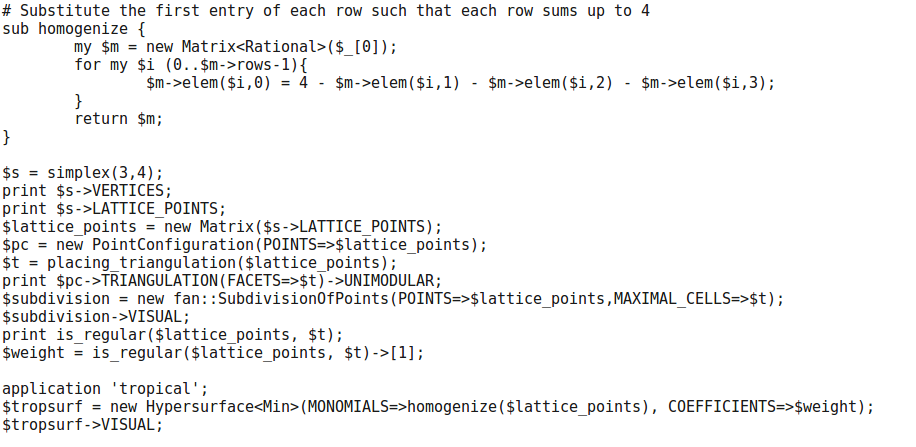# Problem 1

Write a loop that expresses $$x^n+y^n+z^n$$ in terms of elementary symmetric polynomials, for as many positive integers $$n$$ as you can.

## Solution

Following you can find a built in function in Mathematica:

xyzDecomp[n_] := SymmetricReduction[x^n + y^n + z^n, {x, y, z}][]


an example where $$n=5$$:

xyzDecomp


and a plot where the $$x$$ axis is $$n$$ and the $$y$$ axis is how long the computation takes in seconds: